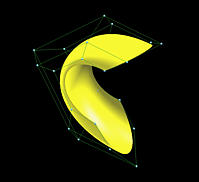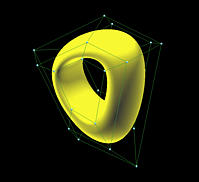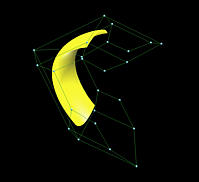# B-spline Surfaces: ConstructionGiven the following information:

1. a set of m+1 rows and n+1 control points pi,j, where 0 <= i <= m and 0 <= j <= n;
2. a knot vector of h + 1 knots in the u-direction, U = { u0, u1, ...., uh };
3. a knot vector of k + 1 knots in the v-direction, V = { v0, v1, ...., vk };
4. the degree p in the u-direction; and
5. the degree q in the v-direction;
the B-spline surface defined by these information is the following: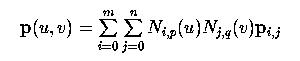where Ni,p(u) and Nj,q(v) are B-spline basis functions of degree p and q, respectively. Note that the fundamental identities, one for each direction, must hold: h = m + p + 1 and k = n + q + 1. Therefore, a B-spline surface is another example of tensor product surfaces. As in Bézier surfaces, the set of control points is usually referred to as the control net and the range of u and v is 0 and 1. Hence, a B-spline surface maps the unit square to a rectangular surface patch.

The following figure shows a B-spline surface defined by 6 rows and 6 columns of control points.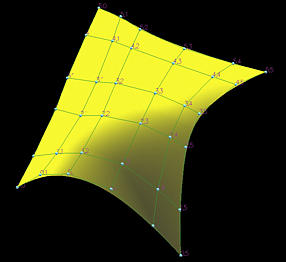The knot vector and the degree in the u-direction are U = { 0, 0, 0, 0.25, 0.5, 0.75, 1, 1, 1 } and 2. The knot vector and the degree in the v-direction are V = { 0, 0, 0, 0, 0.33, 0.66, 1, 1, 1, 1 } and 3.### Basis Functions

The coefficient of control point pi,j is the product of two one-dimensional B-spline basis functions, one in the u-direction, Ni,p(u), and the other in the v-direction, Nj,q(v). All of these products are two-dimensional B-spline functions. The following figures show the basis functions of control points p2,0, p2,1, p2,2, p2,3, p2,4 and p2,5.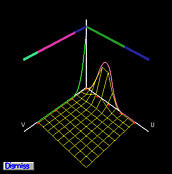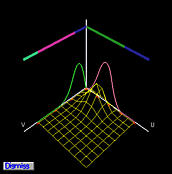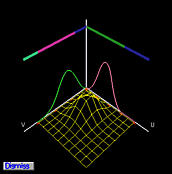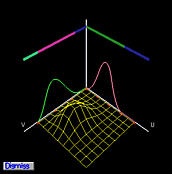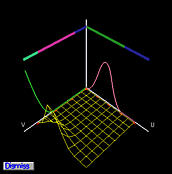The two-dimensional basis functions are shown as wireframe surfaces. Since the control points are on the same row, the basis function in the u-direction is fixed while the basis functions in the v-direction change. Since B-spline basis functions are in general non-zero only on a few consecutive knot spans (i.e., the local modification scheme), the two-dimensional B-spline basis functions are non-zero on the product of two knot spans on which at least one one-dimensional basis function is non-zero. This fact is shown in the above figures clearly.### Clamped, Closed and Open B-spline Surfaces

Since a B-spline curve can be clamped, closed or open, a B-spline surface can also have three types in each direction. That is, we could ask to have a B-spline surface clamped in the u-direction and closed in the v-direction. If a B-spline is clamped in both directions, then this surface passes though control points p0,0, pm,0, p0,n and pm,n and is tangent to the eight legs of the control net at these four control points. If a B-spline surface is closed in a direction, then all isoparametric curves in this direction are closed curves and the surface becomes a tube. If a B-spline surface is open in both directions, then the surface does not pass through control points p0,0, pm,0, p0,n and pm,n. This set of notes only concentrates on B-spline surfaces clamped in both directions. The following figures show three B-spline surfaces clamped, closed and open in both directions. All three surfaces are defined on the same set of control points; but, as in B-spline curves, their knot vectors are different.